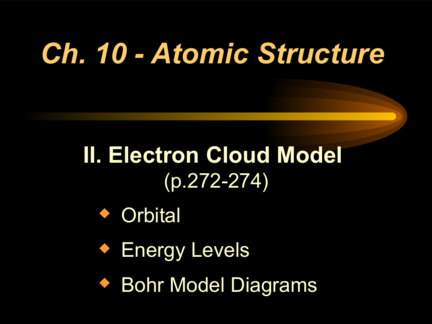# Atomic Structure: What is the Electron Cloud Model?Contributed by:The modern model is also commonly called the electron cloud model. That's because each orbital around the nucleus of the atom resembles a fuzzy cloud around the nucleus, like the ones shown in the Figure below for a helium atom.
1. Ch. 10 - Atomic Structure
II. Electron Cloud Model
(p.272-274)
 Orbital
 Energy Levels
 Bohr Model Diagrams
2. A. Orbital
 Region where there is 90% probability of
finding an electron.
 Can’t pinpoint the
location of an
electron.
 Density of dots
represents degree of
probability.
3. A. Orbital
 Orbitals have different shapes.
4. B. Energy Levels
 Electrons can only exist at
certain energy levels.
 Low energy levels are
close to the nucleus.
 Each energy level (n) can
hold 2n2 electrons.
5. C. Bohr Model Diagrams
 Simplified energy levels using Bohr’s
idea of circular orbits.
Can replace with:
e- 3p
Atomic #: 3 4n
e-
Mass: 7
n np n
pn p Maximum e-
# of p: 3 Level 1 2e-
# of e: 3 e- Level 2 8e-
# of n: 4 Level 3 18e-
Level 4 32e-
6. C. Bohr Model Activity
 Choose a number between 1 & 18.
 Find your element by the atomic number you picked.
 Draw a Bohr Model diagram for your element on your marker board.
• Round off the mass listed on the table and subtract the atomic # to find the # of neutrons.
• Abbreviate the # of ‘p’ and ‘n’ in the nucleus.
 Have a partner check your drawing.
 Repeat with a new element.